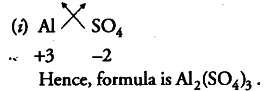# Calculate the molar mass of \$C{{O}_{2}}\$

(i) Write the formula of the compound formed by the ions Al3+ and \$S{ O }{ 4 }^{ 2- }\$
(ii) Calculate the molar mass of \$C{{O}
{2}}\$.(ii) Molar mass of C{{O}_{2}} = 12 + 2 x 16 = 44 u.